# Measurable mapping

(diff) ← Older revision | Latest revision (diff) | Newer revision → (diff)
A mappingof a measurable spaceto a measurable space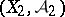such that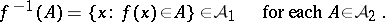In the case where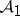is a-algebra andis the real line with the-algebra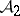of Borel sets (cf. Borel set), the concept of a measurable mapping reduces to that of a measurable function (however, whenis only a-ring, the definition of a measurable function is usually modified in accordance with the requirements of integration theory). The superposition of measurable mappings is measurable. Ifandare rings andfor eachin some class of setssuch that the ring generated by it is the whole of, thenis measurable. The analogous assertions hold in the case of-rings, algebras and-algebras. If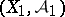andare topological spaces with the-algebras of Borel sets, then every continuous mapping fromto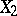is measurable. Letbe a topological space, letbe the-algebra of Borel sets and letbe a finite non-negative regular measure on(regularity means that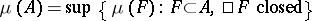). Suppose further thatis a separable metric space,is the-algebra of Borel sets, and letbe a measurable mapping from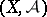to. Then for anythere is a closed subset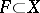such that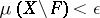andis continuous on(Luzin's theorem).